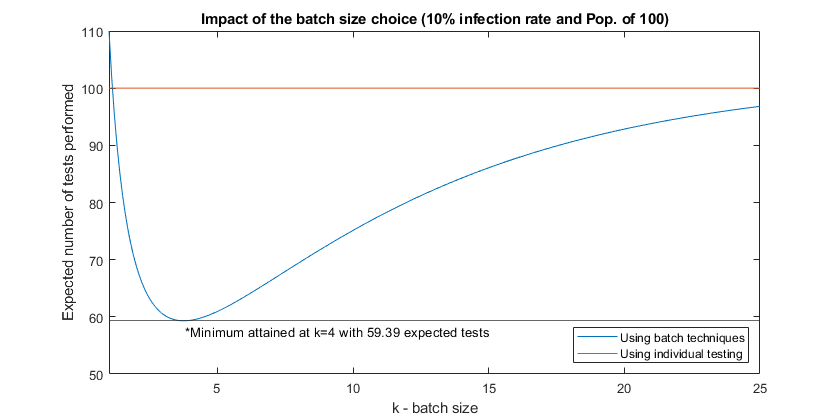## Our Model

### Context

During WWII, R. Dorfman proposed the use of batch testing techniques as a response to the low availability of tests for massive testing.

During the recent CoVid-19 crisis, many researches brought to light the need of massive testing to fight and mitigate the disease consequences (See here or here).

However, some articles, like the ones posted on Washington Post or by French leading Engineering School École Polytechnique, lack in providing the mathematical foundation and tools to define the size of an ideal batch to use when everyone should be effectively tested. Both examples in the articles simply suggest as example to use a batch of 50 individuals, namely, to set a group size as the inverse of the infection probability. Both approaches lack some deeper mathematical analysis that could help find the best batch size.

### Our approach

Mathematically we can demonstrate that the ideal batch size will be dependent on the probability of infection for a given population, thus a fixed batch size is usually sub-optimal. Secondly, it is also clear that the approximation of the inverse of the infection probability is valid only for low values of that same probability, and therefore not applicable to any probability values, or in cases where we will not retest the elements of a group that tested positive.

### Assumptions

• A member of a population is defined as part of the healthy or infected group by a random variable with a known probability distribution;
• Those random variables are assumed independent and identically distributed (iid) among all members of the population;
• If a group tests negative no further testing is done;
• If a group tests positive, it implies that all its members will have to be individually tested.

### Definitions

• $N$ – Total Population;
• $p$ – Infection probability, i.e. probability of a member being infected;
• $k$ – Batch size, i.e. number of members to be included in the same group test.

### Objective

Find the value of $k$ that provides the minimum expected number of tests required to test a population of size $N$ with a probability of infection $p$.

### Methodology

Consider a batch with $k$ individual samples and test it:

• If the test is negative, it means all individuals are negative: $1$ test is enough;
• If the test is positive, it means that at least 1 person is infected: $k + 1$ tests are needed.
(all individual tests to find the infected member(s) + the first initial test)

Thus, for a given batch of $k$ members the test should be:

• Negative with a probability of $(1-p)^k$;
• Positive with a probability of $(1-(1-p)^k)$.

We can finally write that, as an approximation, the expected number of tests needed for $\frac{N}{k}$ groups will be the summation of the total tests, that is given by the sum of the probability of the test be negative times the tests needed (written above to be equal to $1$) and the probability of the test be positive times the tests needed (written above to be equal to $k + 1$):

$\mathbb{E}[tests]=\sum_{i=0}^{N/k} \left[ 1 \times (1-p)^k + (k+1) \times (1-(1-p)^k)\right]$

### Example